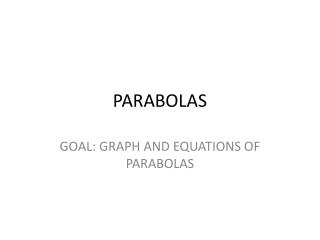DownloadDownload PresentationPARABOLAS

# PARABOLAS

Télécharger la présentation## PARABOLAS

- - - - - - - - - - - - - - - - - - - - - - - - - - - E N D - - - - - - - - - - - - - - - - - - - - - - - - - - -
##### Presentation Transcript

1. PARABOLAS GOAL: GRAPH AND EQUATIONS OF PARABOLAS

2. DEFINITIONS • Focus – lies on the axis of symmetry • Vertex – lies halfway between the focus and the directrix • Directrix – perpendicular to the axis of symmetry • Parabola – the graph of a quadratic equation (u-shaped)

3. STANDARD EQUATION OF A PARABOLA WITH VERTEX AT (0,0) • Equation - X2 = 4py Y2 = 4px • Focus – (0,p) (p,0) • Directrix - Y = -p X = -p • Axis of • Symmetry – Vertical (X=0) Horizontal (y=0)

4. EXAMPLE 1 • Identify the focus and the directrix of the parabola given by X=3/4y2. Draw the parabola.

5. EXAMPLE 2 • Write an equation of the parabola shown below

6. PARABOLAS IN THE REAL WORLD • You will find five pictures on the internet that displays a parabola and print them out. • We will use them for discussing and graphing.